Next: Analyzing univariate polynomials Up: ALIAS-C++ Previous: Analyzing systems of equations

• La page de J-P. Merlet
• La page Présentation de HEPHAISTOS
• La page "Présentation" de l'INRIA

Subsections

# Introduction

The purpose of this chapter is to describe tools which can be used to analyze either one trigonometric equation in one variable or a system of trigonometric equations. These tools may be used either to improve the efficiency of the solving algorithm described in the previous chapter or even to avoid calling them if some constraints are imposed on the location of the solution.

# Number of roots of trigonometric equation

The purpose of this section is to present an algorithm which enable to determine how many roots has an equation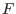in the unknown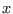of the form: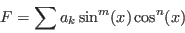(4.1)

with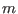in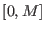and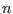in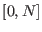, both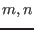being integers, andin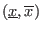.

## Mathematical background

We use the half angle tangent substitution. If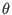is the unknown we define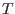as: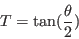Then we have: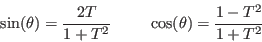Note that the change of variable is not valid if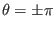. In that case it will be preferable to define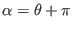and to transform the initial into an equation in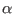. Then the change of variable may be applied.

Using the above relation any trigonometric equation can be transformed into a polynomial equation which is analyzed using the tools of chapter 5.

It remains to define an interval for angles that we will denote an angle interval. The element of an angle interval is usually defined between 0 and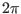(although in most of the following procedures any value can be used when not specified: internally the element of the angle interval are converted into value within this range). A difference between numbers interval (INTERVAL) and angle interval is that the lower bound of an angle interval may be larger than the upper bound. Indeed the order in an angle interval is important: for example the angle intervals [0,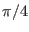] and [,0] are not the same.

## Implementation

The purpose of the implementation is first to convert the trigonometric equation in sine and cosine of: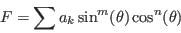(4.2)

into a polynomial in either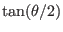or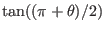. A first procedure return an upper bound of the degree of the resulting polynomial:

int Degree_Max_Convert_Trigo_Interval(int n,VECTOR &A,INTEGER_VECTOR &SSin,INTEGER_VECTOR &CCos);
int Degree_Max_Convert_Trigo_Interval(int n,INTEGER_VECTOR &A,
INTEGER_VECTOR &SSin,INTEGER_VECTOR &CCos);

with:
• n: number of terms of the equation
• A: the coefficients of each term which may be either real or integer
• Ssin: the sine power of each term
• Ccos: the cosine power of each term
Then we may use a procedure which compute the coefficients of the polynomial equation using the following procedures:

VOID Convert_Trigo_Interval(int n,VECTOR &A,INTEGER_VECTOR &SSin,
INTEGER_VECTOR &CCos,VECTOR &Coeff,int *degree);
VOID Convert_Trigo_Interval(int n,INTEGER_VECTOR &A,INTEGER_VECTOR &SSin,
INTEGER_VECTOR &CCos,INTEGER_VECTOR &Coeff,int *degree);
VOID Convert_Trigo_Interval(int n,INTERVAL_VECTOR &A,INTEGER_VECTOR &SSin,
INTEGER_VECTOR &CCos,INTERVAL_VECTOR &Coeff,int *degree);

with:
• n: number of terms of the equation
• A: the coefficients of each term which may be either real or integer
• Ssin: the sine power of each term
• Ccos: the cosine power of each term
• Coeff: the coefficients of the polynomial
• degree: the final degree of the polynomial
The previous procedures use the substitution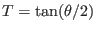which is not valid for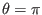. In that case we may use instead the substitution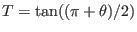and the coefficient of the resulting polynomial may be determined using the following procedures:

VOID Convert_Trigo_Pi_Interval(int n,VECTOR &A,INTEGER_VECTOR &SSin,
INTEGER_VECTOR &CCos,INTEGER_VECTOR &Coeff,int *degree);
VOID Convert_Trigo_Pi_Interval(int n,INTEGER_VECTOR &A,INTEGER_VECTOR &SSin,
INTEGER_VECTOR &CCos,INTEGER_VECTOR &Coeff,int *degree);
VOID Convert_Trigo_Pi_Interval(int n,INTERVAL_VECTOR &A,INTEGER_VECTOR &SSin,
INTEGER_VECTOR &CCos,INTEGER_VECTOR &Coeff,int *degree);

Similar procedures exists for interval trigonometric equations i.e. equations where the coefficients A are intervals. In that case degree will no more an integer but an INTERVAL which indicate the lowest and highest degree of the resulting polynomial. In some case the number of roots of the trigonometric equation may exceed the degree of the equivalent polynomial. For example the equation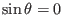has the roots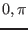while the degree of the equivalent polynomial is only 1. In all cases the total number of roots of the trigonometric equation will never exceed the degree+2.

Having determined the equivalent polynomial you my use the tools described in section 5 for determining the number of roots of the trigonometric equation. But you still have to manage the search interval. The following procedure is able to determine this search interval and to determine the number of roots of the trigonometric equation:


int Nb_Root_Trigo_Interval(int n,VECTOR &A,INTEGER_VECTOR &SSin,
INTEGER_VECTOR &CCos,REAL Inf,REAL Sup)

with:
• n: number of terms of the equation
• A: the coefficients of each term which may be either real or integer
• Ssin: the sine power of each term
• Ccos: the cosine power of each term
• Inf: the lower bound of the search interval
• Sup: the upper bound of the search interval
On success this procedure returns a number greater or equal to 0 and returns -1 if it has failed. Failure occurs either if the equation is equal to 0 or if Sturm method failed to determine the number of roots of the equivalent polynomial equation (this will happen if Inf or Sup are exact root of the equation).

## Example

Consider the equation: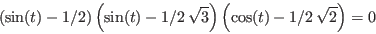which has as roots: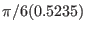,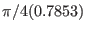,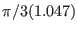,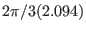,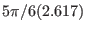,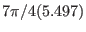. This equation is equivalent to: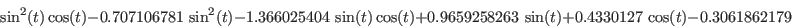The A, SSin, CCos vectors have the following values:
 A SSin CCos 1 2 1 -0.7071067810 2 0 -1.366025404 1 1 0.9659258263 1 0 0.4330127020 0 1 -0.3061862179 0 0
If Inf=0 and Sup=0.8 the procedure indicates that there are two roots corresponding to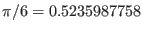and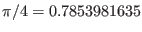.

# Bound on the roots of trigonometric equation

The purpose of this section is to present an algorithm which enable to determine bounds on the roots of an equationin the unknownof the form:(4.3)

withinandinandin.

## Implementation

This procedure return angle intervals included in the range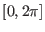which contain the roots of the trigonometric equation.

int Bound_Root_Trigo_Interval(int n,VECTOR &A,INTEGER_VECTOR &SSin,
INTEGER_VECTOR &CCos,int *nbsol,VECTOR &Inf,VECTOR &Sup);

with:
• n: number of terms of the equation
• A: the coefficients of each term which may be either real or integer
• Ssin: the sine power of each term
• Ccos: the cosine power of each term
• nbsol: the number of angle intervals which are returned by the procedure (at most 4)
• Inf: the lower bound of the returned angle interval
• Sup: the upper bound of the returned angle interval
Note that the size of Inf, Sup should be 4 at least. In case of failure the procedure return -1, 1 on success.

### Example

Consider the equation:which has as roots:,,,,,. The procedure returns 2 angle intervals: [0.517117,2.62532], [5.49664,5.50734].

# Utilities for trigonometric equation

## Inclusion in an angle interval

The procedure:


int Angle_Ok_Interval(double angle,double b1,double b2);

return 1 if the angle angle belongs to the angle interval [b1,b2], 0 otherwise. The angle angle,b1, b2 should have a value within [0,].

## Distance between two angles

The procedure:


double Distance_Angle(double a1,double a2)

return the smallest distance in radian between the two angles a1,a2.

## Generalized inverse trigonometric functions

Assume that we have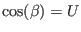where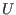is an interval and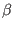should lie in an arbitrary range. The procedure Filtre_Arc_Cos allows to update the range for. It returns -1 isandare incompatible, 0 otherwise. Its syntax is:


int Filtre_Arc_Cos(INTERVAL &U,INTERVAL &beta)

A similar procedure exist for the inverse sine with

The procedure Arc_Cos_Multiple allows to determine all possible ranges for, assuming thatis restricted to a range included in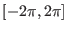::


int Arc_Cos_Multiple(INTERVAL &U,INTERVAL &beta,INTERVAL_VECTOR &BETA)

This procedure returns the number of possible ranges forand their values in BETA.Next: Analyzing univariate polynomials Up: ALIAS-C++ Previous: Analyzing systems of equations